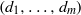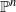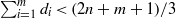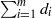HomeCompositio Mathematica

# Spaces of rational curves on complete intersections

Published online by Cambridge University Press:  26 March 2013

## Abstract

We prove that the space of smooth rational curves of degree$e$ on a general complete intersection of multidegree$(d_1, \ldots , d_m)$ in$\mathbb {P}^n$ is irreducible of the expected dimension if$\sum _{i=1}^m d_i \lt (2n+m+1)/3$ and$n$ is sufficiently large. This generalizes a result of Harris, Roth and Starr [Rational curves on hypersurfaces of low degree, J. Reine Angew. Math. 571 (2004), 73–106], and is achieved by proving that the space of conics passing through any point of a general complete intersection has constant dimension if$\sum _{i=1}^m d_i$ is small compared to$n$ .

## MSC classification

Type
Research Article
Information
Compositio Mathematica , June 2013 , pp. 1041 - 1060

## References

[BM96]Behrend, K. and Manin, Yu., Stacks of stable maps and Gromov–Witten invariants, Duke Math. J. 85 (1996), 160.CrossRefGoogle Scholar
[CS09]Coskun, I. and Starr, J., Rational curves on smooth cubic hypersurfaces, Int. Math. Res. Not. 2009 (2009), 46264641.Google Scholar
[Deb01]Debarre, O., Higher dimensional algebraic geometry (Springer, New York, 2001).CrossRefGoogle Scholar
[dJS04]de Jong, A. J. and Starr, J., Cubic fourfolds and spaces of rational curves, Illinois J. Math. 48 (2004), 415450.Google Scholar
[dJS06]de Jong, A. J. and Starr, J., Low degree complete intersections are rationally simply connected, Preprint (2006).Google Scholar
[Del04]Deland, M., Geometry of rational curves on algebraic varieties, PhD thesis, Columbia University (2004).Google Scholar
[Ein86]Ein, L., Varieties with small dual varieties I, Invent. Math. 86 (1986), 6374.CrossRefGoogle Scholar
[FP97]Fulton, W. and Pandharipande, R., Notes on stable maps and quantum cohomology, in Algebraic geometry: Santa Cruz 1995, Proceedings of Symposia in Pure Mathematics, vol. 62 eds Kollár, J., Lazarsfeld, R. and Morrison, D. (American Mathematical Society, Providence, RI, 1997).Google Scholar
[HRS04]Harris, J., Roth, M. and Starr, J., Rational curves on hypersurfaces of low degree, J. Reine Angew. Math. 571 (2004), 73106.Google Scholar
[Har77]Hartshorne, R., Algebraic geometry, Graduate Texts in Mathematics, vol. 52 (Springer, New York, 1977).CrossRefGoogle Scholar
[Kle74]Kleiman, S. L., The transversality of a general translate, Compositio Math. 28 (1974), 287297.Google Scholar
[Kol96]Kollár, J., Rational curves on algebraic varieties, Ergebnisse der Mathematik und ihrer Grenzgebiete, 3. Folge, vol. 32 (Springer, Berlin, 1996).CrossRefGoogle Scholar
[Mat86]Matsumura, H., Commutative ring theory, Cambridge Studies in Advanced Mathematics, vol. 8 (Cambridge University Press, Cambridge, 1986).Google Scholar
[Mum70]Mumford, D., Abelian varieties, Tata Institute of Fundamental Research Studies in Mathematics, vol. 5 (Oxford University Press, Oxford, 1970).Google Scholar
[Sta04]Starr, J., The Kodaira dimension of spaces of rational curves on low degree hypersurfaces, Preprint (2004).Google Scholar
[Vak00]Vakil, R., The enumerative geometry of rational and elliptic curves in projective space, J. Reine Angew. Math. 529 (2000), 101153.Google Scholar

### Full text views

Full text views reflects PDF downloads, PDFs sent to Google Drive, Dropbox and Kindle and HTML full text views.

Total number of HTML views: 0
Total number of PDF views: 108 *
View data table for this chart

* Views captured on Cambridge Core between September 2016 - 20th January 2021. This data will be updated every 24 hours.

Access
Hostname: page-component-76cb886bbf-m9qpn Total loading time: 0.542 Render date: 2021-01-20T14:28:06.092Z Query parameters: { "hasAccess": "1", "openAccess": "0", "isLogged": "0", "lang": "en" } Feature Flags: { "shouldUseShareProductTool": true, "shouldUseHypothesis": true, "isUnsiloEnabled": true, "metricsAbstractViews": false, "figures": false, "newCiteModal": false }

# Send article to Kindle

Note you can select to send to either the @free.kindle.com or @kindle.com variations. ‘@free.kindle.com’ emails are free but can only be sent to your device when it is connected to wi-fi. ‘@kindle.com’ emails can be delivered even when you are not connected to wi-fi, but note that service fees apply.

Find out more about the Kindle Personal Document Service.

Spaces of rational curves on complete intersections
Available formats
×

# Send article to Dropbox

To send this article to your Dropbox account, please select one or more formats and confirm that you agree to abide by our usage policies. If this is the first time you use this feature, you will be asked to authorise Cambridge Core to connect with your <service> account. Find out more about sending content to Dropbox.

Spaces of rational curves on complete intersections
Available formats
×

# Send article to Google Drive

To send this article to your Google Drive account, please select one or more formats and confirm that you agree to abide by our usage policies. If this is the first time you use this feature, you will be asked to authorise Cambridge Core to connect with your <service> account. Find out more about sending content to Google Drive.

Spaces of rational curves on complete intersections
Available formats
×
×